## Graphing Calculator

### What's new in Version 4.1 for macOS

• 64-bit binary
• Sliders for point radius and line width
• Select vector and matrix elements by subscript
• Allows slider variables in set definitions
• Support arrows and complex points on right hand graph pane in split views
• Support trackpad for zoom on 2D graph views
• Removed 4D graphing

### What was new in Version 3.0

• Text comments in documents
• Printing
• Multiple document windows
• Save as web page
• Save as RTF for export to word processors
• 3.0 acts as a helper application for GC documents posted to the web

### What was new in Version 2.7

#### Tables

You can enter tables of data, numerically transform the data, and graph data in two and three dimensions.#### Surfaces of revolution

You can rotate a curve around an arbitrary axis to generate a surface in three dimensions.#### 3D Ordinary Differential Equations

You can graph ODEs in three dimensions.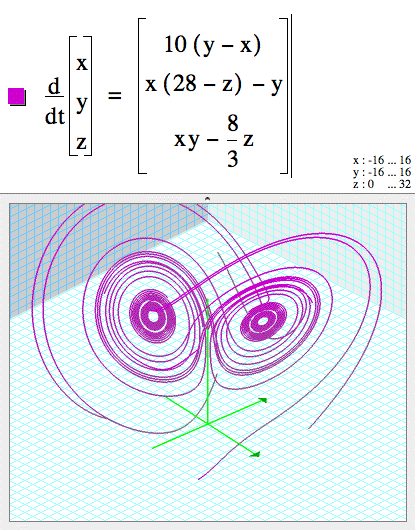You can specify initial conditions for ODEs by giving starting points on surfaces.#### Tube plots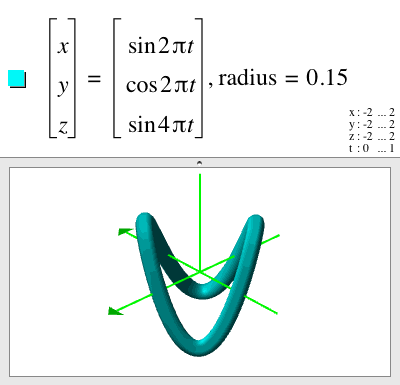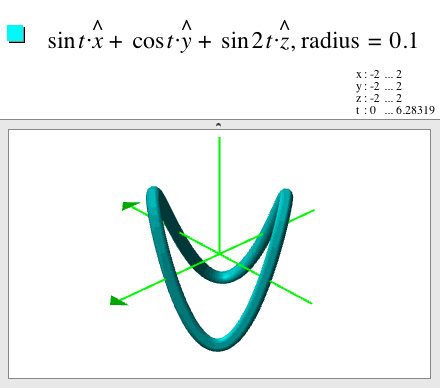#### Numeric integration#### Binomial coefficient#### Products#### Matrix operations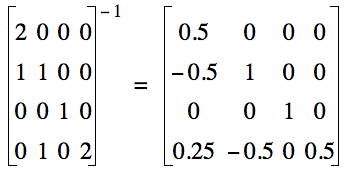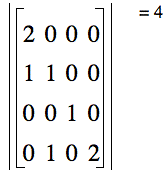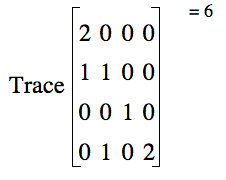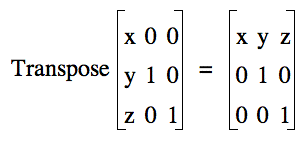#### Complex Conjugate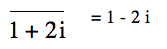#### Logical expressions#### Vector fields

Vector fields can be graphed on surfaces.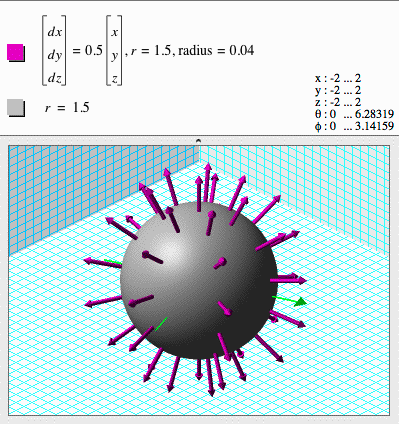#### Parameter sets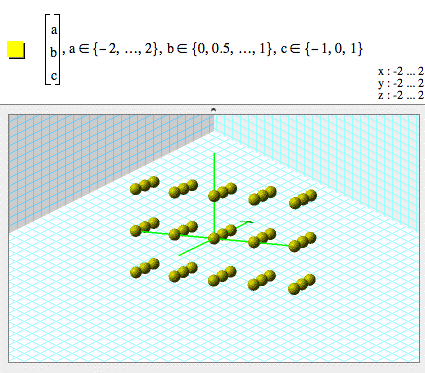#### 2D Vectors#### 3D Vectors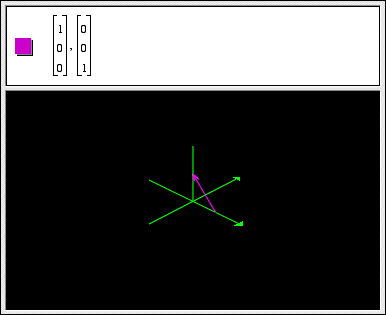#### Coordinate Transformations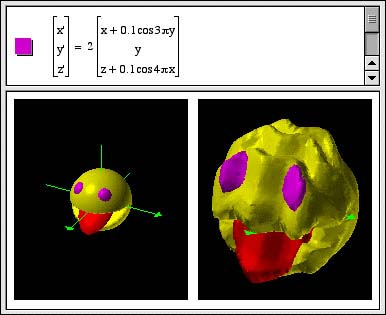#### Complex number arithmetic

You can use Graphing Calculator 3.1 as a calculator for complex numbers. For example:#### Complex function parametric curves in 2D

A complex function of a real parameter, t, specifies a curve in 2D. For example:This is a shorter way of writing the parametric equation:You can also use z as shorthand for x + iy. For example: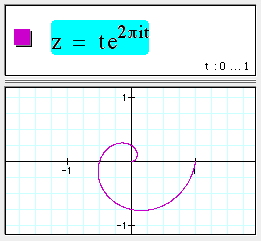#### Complex function parametric curves in 3D

A complex function of a real parameter, z, specifies a curve in 3D. Observe that this is the same complex function as in the 2D example above, but here the parameter z is used as the third 3D coordinate.This is similar to writing the parametric equations: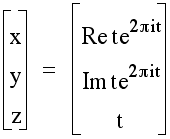#### Parametric curves in 4D

You can also write parametric equations for curves in four dimensions by specifying functions (which have real values) for each of the four spatial coordinates x, y, u, and v. For example:#### Complex function surfaces in 3D

Using z as shorthand for x + iy, you can graph 3D surfaces representing complex functions. For example: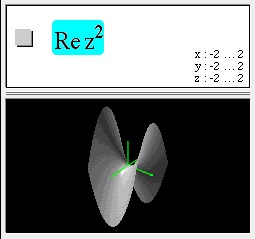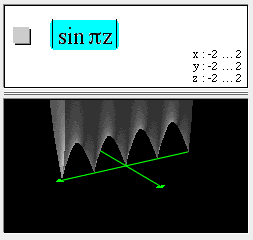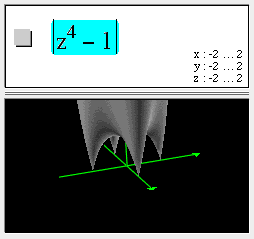In this example, observe the four zeros at +1, -1, +i, and -i.

#### Complex function surfaces in 3D with color coding

Using z as shorthand for x + iy, you can graph 3D surfaces representing complex functions. The height of the surface represents the magnitude of the complex function. The phase of the complex function is encoded in the color of the surface. For example: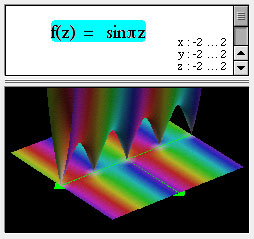#### Complex function surfaces in 4D

A complex function f(x + iy) depends on two real parameters x & y and has two components, Re f, and Im f. We can render this as a surface in 4 spatial dimensions with coordinates:
(x, y, Re f(x+ iy), Im f(x + iy)) and then project this surface to the screen in a manner similar to the way we project 3-D surfaces onto a 2-D screen. Note that in the following, z=x+iy and w=u+iv.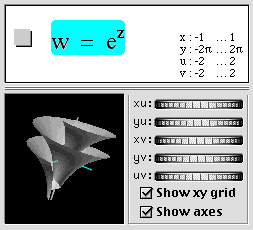#### Interactive complex parameters

In the following example, you can drag the purple dot, which moved the square grid along with it. The blue grid is the map of the square grid under the complex function f(z).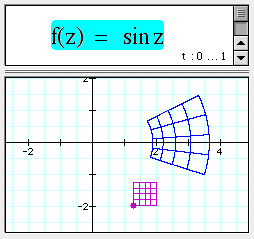#### Color maps

You can specify the color at each point in the place by giving functions for either the (red, green, blue) or the (hue, saturation, value) components of the color.#### Point plots

In the following example, as n varies, the point traces out a circle:#### Vertex lists in 4D

The following shows a hypercube in four dimensions specified by writing the 32 edges between its 16 vertices.#### Parametric surfaces in 4D

This is the parametrization for a flat torus in 4D. It is the product of an x-y circle (sin u, cos u), and a u-v circle (sin v, cos v). (The four 4D coordinate axes are x, y, u & v. The letters u & v are also used separately for the surface parametrization. This is confusing as u and v must be interpreted differently depending on context.)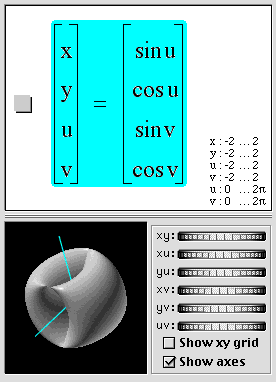#### New functions

The min() function returns the minimum of its arguments.The max function returns the maximum of its arguments.mod(a,b) return the remainder of a divided by b.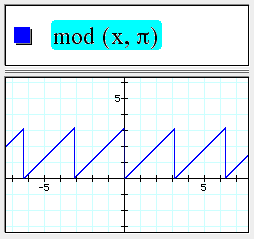clamp(x,a,b) pins x to the range [a,b].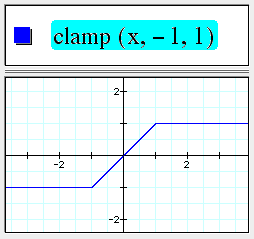#### Text expressions in coordinate ranges#### Direction fields

Type command-option-d to draw unit vectors in a vector field.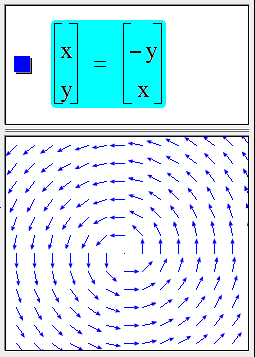#### Examples menu and sample documents

Version 3.1 ships with example and template documents which are also accessible from the new Examples menu.

#### Background Color

The preferences dialog now gives control over the background used with 3D objects.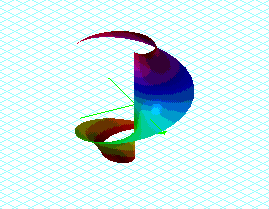#### Defining variables and functions(You must type "f control-9 x" to distinguish f(x) as a function.)

#### Copy and Paste formulas as plain text

Copy as Text on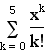produces sum(x^k/k!,k=0,5).

#### Equations line-break and scroll#### 1st order 2D ordinary differential equations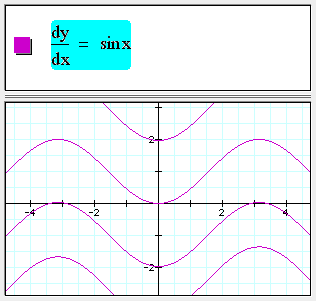#### Fractional powers

Fractional powers of negative numbers are handled properly now.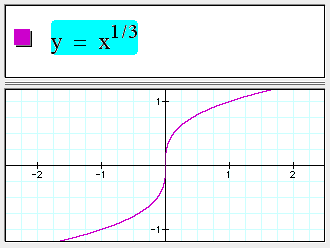#### Bessel functions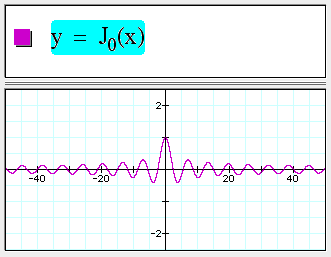#### Floor and ceiling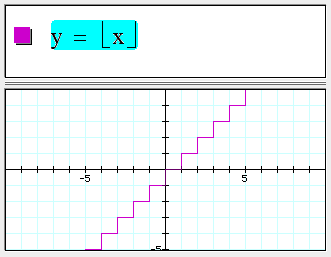#### Piecewise defined functions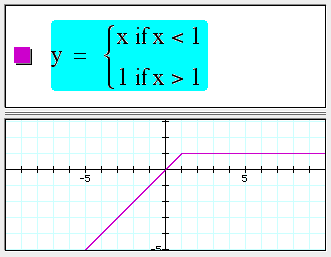#### Div, grad, and curl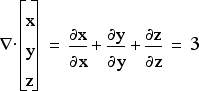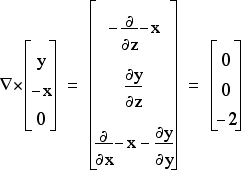#### Vector dot and cross products#### Summations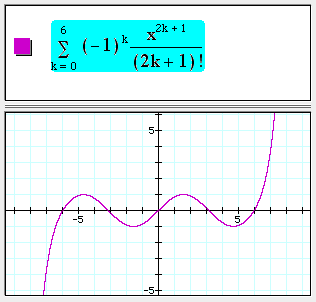#### Substitutions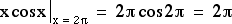#### New releases

If you would like to help test pre-release development versions of our products, subscribe to our mailing lists here.